# Community

Blog Events Webinars Tutorials Forum
×
Community Blog Learning about Distributed Systems - Part 21: Cost-based Optimization

# Learning about Distributed Systems - Part 21: Cost-based Optimization

Part 21 of this series focuses on why there is a CBO and how it is implemented.

## Close to the Essence of Performance Optimization

In the previous article, we briefly introduced the rule-based optimization RBO and finally mentioned that many problems cannot be solved by RBO because it is summarized from experience.

The fundamental reason is that the experience induction is flawed, and it is difficult to cover all aspects.

For example, if the value distribution is uneven, a full table scan is better than index querying.

For example, in the case of multi-table join, it is impossible to summarize a fixed rule to which table is better to put first.

In short, the abstract RBO is too indirect to be practical, so we need a more direct and detailed optimization method.

Let's consider a few basic questions:

What are the goals of performance optimization?

• Shorter execution time
• Lower latency
• Greater throughput
• Less resource usage

What is the focus of performance optimization?

• In distributed programs, we focus on Shuffle because it involves plenty of IO, and IO is the biggest drag on performance.
• In SQL Join, we focus on Shuffle and consider Match because it involves plenty of IO and computing.
• In Spark ML, we cache data to avoid repeated iterative computing because the computing is too much.

It seems the more you think about it, the more complicated it is, but that isn't all. There is a saying:

If you cannot measure it, you cannot optimize it.

How can we measure performance?

• In conventional algorithms, we measure an algorithm by its time complexity and space complexity.
• How can we measure an algorithm in distributed computing or SQL?

What about time complexity and space complexity? It seems to be enlightening.

Time complexity can correspond to computing, and space complexity is the same as memory occupancy.

As such, we can similarly design the performance measurement of distributed programs from the perspective of resource occupancy.

So, the question becomes – what system resources would a distributed program use?

Let's take a look at the main resources that a program will use on a computer:

• CPU
• Memory
• IO
• Disk IO
• Network IO

Expand to multiple nodes under distributed programs. Considering the topic of this article and our recent focuses, Hive and Spark, which both process a large amount of data on HDFS, HDFS IO needs to be introduced because reading data from HDFS may be read from local disks or pulled from the network and cannot be determined in advance.

On the other hand, it is difficult to compute memory occupancy in advance. It is easy to understand. For example, Shuffle and Join mentioned earlier may adopt different algorithms for different data volume and parameter settings, and there are operations (such as spill in the process). In addition, the influence of actual data on compression ratio and other factors all make it difficult to accurately estimate memory usage.

Based on these considerations, we focus on these aspects of resource consumption for distributed programs, including SQL:

• CPU
• IO
• Disk IO
• Network IO
• HDFS IO

The purpose of performance optimization is to reduce the consumption of these aspects. The effect of performance optimization is also measured by these attributes.

## Define Cost Model

Several attributes that can measure performance are listed above, but they still need to be quantified into metrics before they can be computed.

Taking Hive as an example, it is not difficult to define some parameters. Spark SQL is similar to Hive.

Unit Consumption:

• Hr is the consumption of reading 1 byte from HDFS in nanoseconds.
• Hw is the consumption of writing 1 byte to HDFS in nanoseconds.
• Lr is the consumption of reading 1 byte from the local disk in nanoseconds.
• Lw is the consumption of writing 1 byte to the local disk in nanoseconds.
• NEt is the consumption of 1 byte of network transmission in nanoseconds.
• CPUc is the CPU consumption of the compare operation in nanoseconds.

Statistical Data:

• T(R) - Number of rows
• Tsz - The average size of each row
• V(R, a) - The number of distinct counts in column a of table R

If the metric is available, there must be a formula to produce a result that can be directly compared before it can be used.

As mentioned in the previous article, SQL parsing and RBO optimization are based on AST.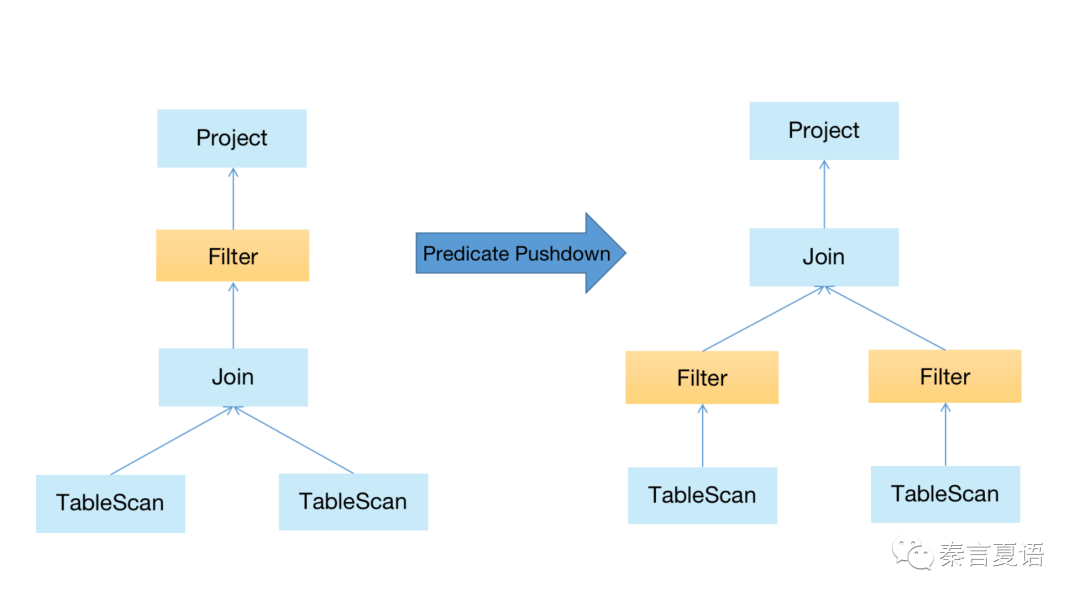As shown in the example above, since RBO can be optimized based on AST, can we design our resource consumption formula based on AST?

The AST is composed of a series of operations. If we can compute the resource consumption of each operation, then, in theory, if we add them up, it is the total consumption of AST, which is the consumption of SQL.

So, the question becomes – how can we compute the consumption of each operation?

It is no trouble. After all, SQL is a declarative language and supports limited operations, just define all of them once.

In addition to CPU and IO, we consider the number of rows for the cost. The reason will be discussed later.

Let's start with the simplest one, the consumption of Table Scan. Scan a table. It is operated by HDFS IO with no CPU involved. The number of rows is the number of rows in the table.

• CPU Cost = 0
• IO Cost = Hr T(R) Tsz
• Number of Rows = T(R)

Let's continue with a slightly more complicated one. Two tables do the consumption of Map Join. First, distribute the small tables to each mapper, build a HashTable, and perform Hash Join.

• CPU Cost = (T(R2)+(T(R1)+T(R2)))* CPUc
• IO Cost = T(R2) Tsz NEt * number of mappers
• Number of Rows=Join Cardinality

Finally, look at an example of a Filter. Filtering data is pure CPU overhead because IO overhead has been computed in the previous step of the Filter.

• CPU Cost = T(R) * CPUc
• IO Cost = 0
• Number of Rows=Filter Selectivity * Number of Rows from Child

Like the three examples above, all operations can compute the overhead, and adding up the cost of each operation in AST can get the total overhead.

The total overhead is defined as cost. The optimization method that computes the cost of each plan and then selects the method with the lowest cost is called Cost Based Optimization (CBO).

However, we have listed several different overheads above. Since they have different attributes, how can we add them up? Why do we separate the number of rows?

We find the answer from the source codes.

Let's see how Hive compares the cost of two execution plans: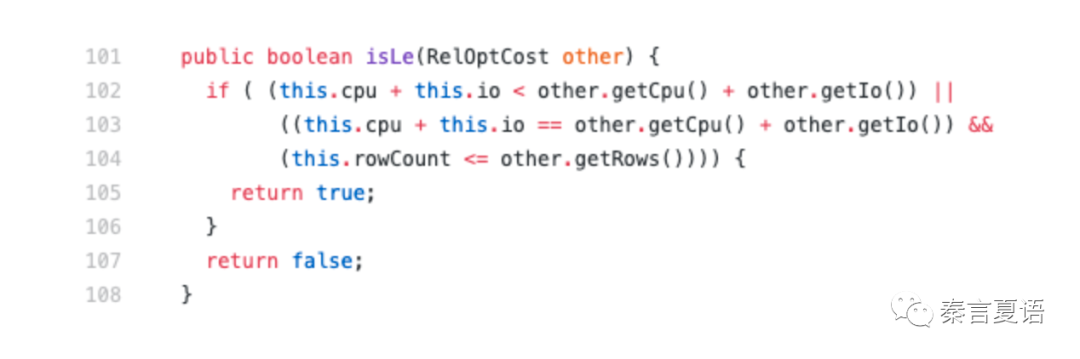Hive thinks CPU and IO are more important, so they should be compared first. However, it is unnecessary to study further between CPU and IO and directly add them together. If the values of CPU+IO of the two execution plans are equal, compare the number of rows.

As we mentioned in the previous article, the Optimizer of Hive uses Apache Calcite, and Calcite compares the costs of the two plans this way: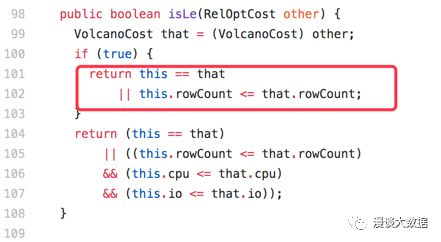Only the number of rows is considered.

Therefore, we can understand why Hive uses this method to compare costs. On the one hand, it is close to the essence of cost. On the other hand, it retains the most basic and traditional cost with the number of rows as the core as a supplement.

It is understandable to use the number of rows to cover the bottom. After all, there are still factors (such as memory) we have not considered or cannot consider, which are not included in the cost. The abstract level of the number of rows is between rules and resource consumption, which is relatively appropriate.

However, adding CPU and IO directly is a bit imprecise. The cost and scarcity of CPU and IO are different in a resource-competitive environment.

Therefore, as a rising star, Spark SQL's cost formula is better than Hive’s.

Earlier, Spark SQL used weight parameters to distinguish the importance between CPU and IO, and the cost formula was designed based on the `weight * CPU Cost + (1-weight) * IO Cost`.

For simplicity, we use `weight * cardinality + (1-weight) * size` and replace CPU and IO with cardinality and size.

It later evolved into the following: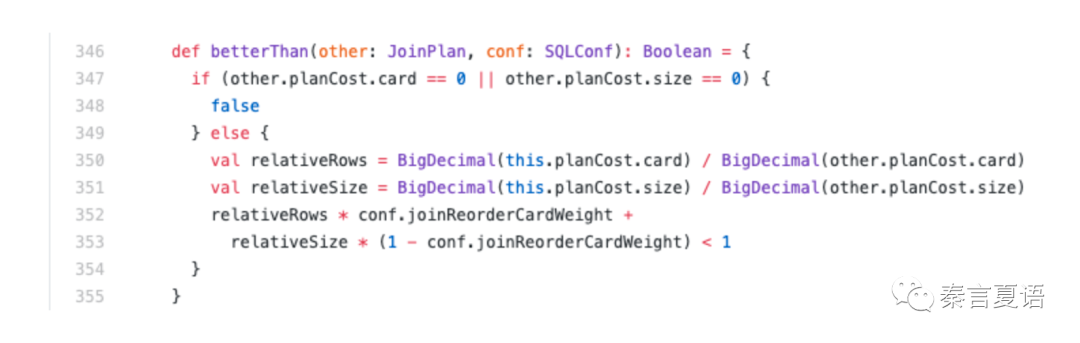In addition to the absolute weight parameters, the relative values of cardinality and size are computed separately, so the method of adding relative absolutes can also avoid mutual influence.

The cost formula is evolving, hoping to strike a balance between rationality and cost as much as possible.

## Collect Statistical Data

As mentioned earlier, when we compute the cost, there are some statistical data in addition to the fixed unit consumption. It is easy to understand. Unit price × Quantity is equal to the total price.

As for these statistical data, since they are directly related to specific data, they need to be collected and computed.

Looking closer, these statistical data can be divided into two types:

• Table-level statistical data (such as the number of rows and size)
• Column-level statistical data (such as the maximum and minimum values)

They provide `analyze` commands for active data collection in mainstream databases, including our focuses, Hive and Spark.

For example, in such a partition of a Hive table, the initial values of `numRows` and `rawDataSize` are both -1, which is unknown.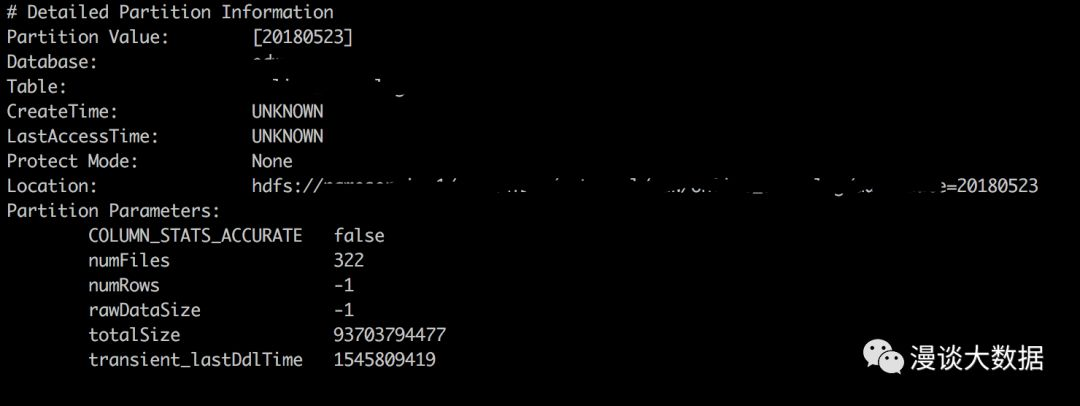Let's perform `analyze table [table_name] partition([partition_expr]) compute statistics;` below and then describe it.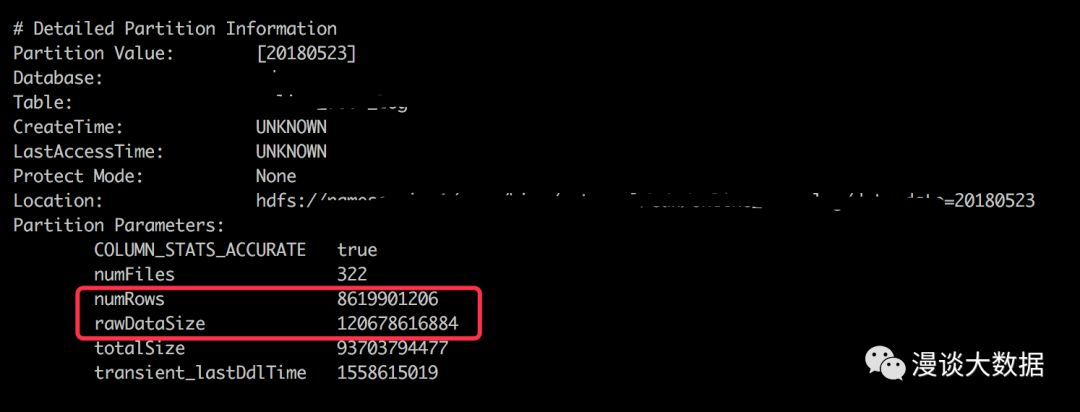`numRows` and `rawDataSize` have real values.

While defining the cost of the Filter operation above, `Number of Rows = Filter Selectivity * Number of Rows from Child`, we encountered a new parameter called `Selectivity`.

`Selectivity` refers to the proportion of filtering, which requires column-level data distribution to be estimated.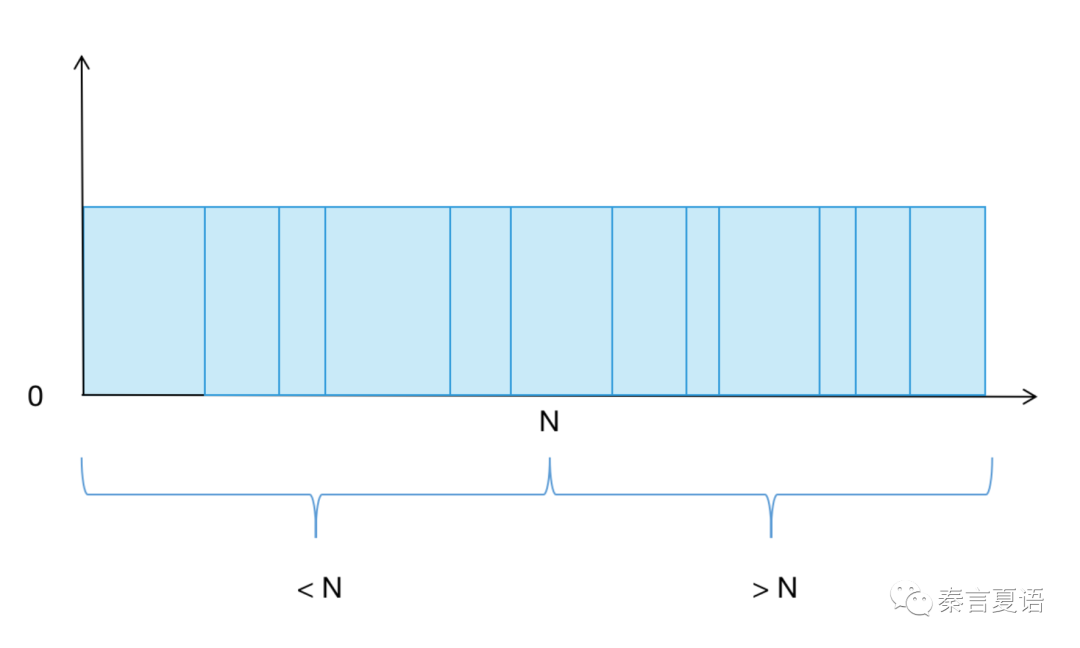The distribution of data is usually recorded using an equi-height map like the one above. Since the equi-height distribution map is more in line with the actual situation than the linear distribution map. It can avoid the estimation error caused by data skew compared with the equi-width map.

For a histogram diagram (like data distribution), you need to collect column-level statistical data first by adding `for columns` after the `analyze` command that just collected table-level statistical data.

Then, the statistical data required by CBO can be collected in advance and saved to the metabase using these two different levels of analyze commands. When the task is executed, it will be directly used as the basis for cost computing.

However, these statistical data need to consume resources to compute. For example, in Hive, MR tasks are executed to compute them.

We need to execute it judiciously and selectively:

• Determine the range as needed. Rarely used tables or columns can be ignored.
• The frequency of updating statistical data does not need to be more frequent than updating data.
• Try to compute statistics during off-peak hours to avoid affecting business applications.

## See the Power of CBO

Having said all of the above, it is not down-to-earth enough. Whether the effect of CBO is magical or not needs to be tested in practice.

As we said earlier, Join Order is beyond RBO, and CBO is needed. Let's take this example to illustrate the power of CBO.

First of all, from the introduction to the principle of join in the previous article, we can know that when multiple tables are joined, the sequence has a huge impact on performance.

Let’s take three tables A join B join C as an example. The following chart shows the number of rows in each table and the join results.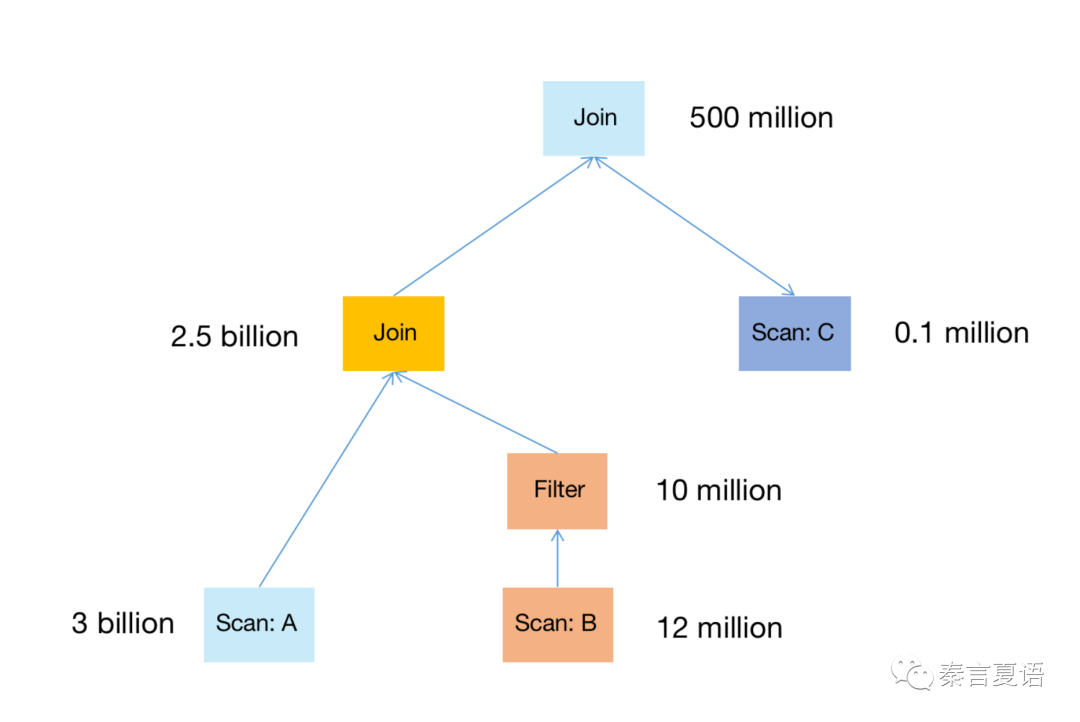After CBO optimization, the join order will be adjusted: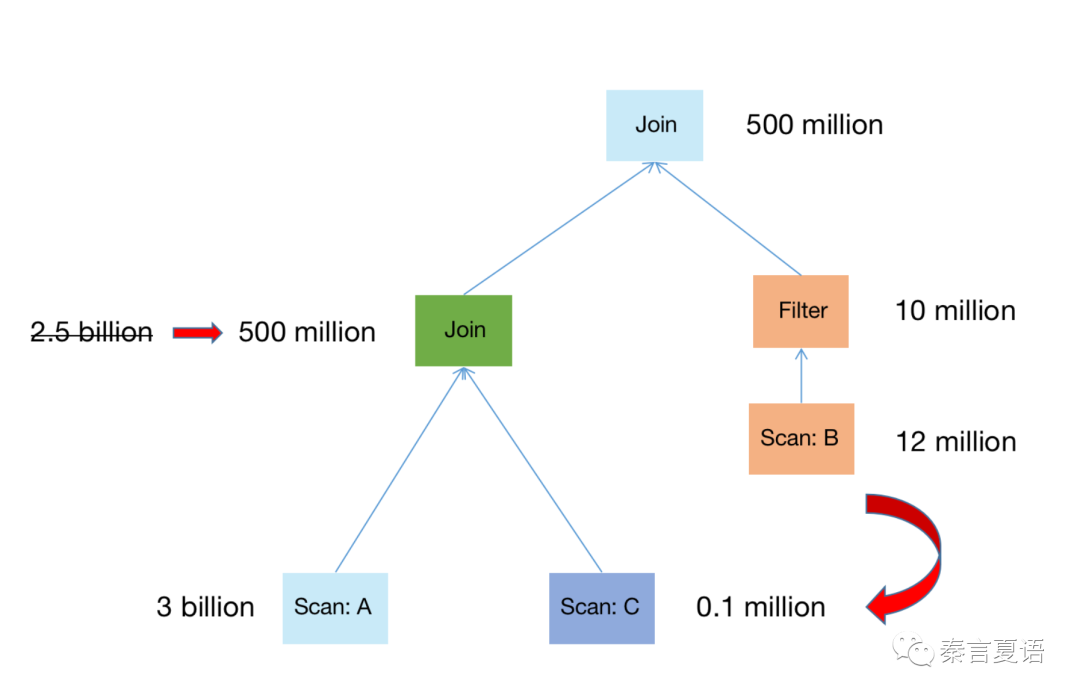After we adjusted the order, which means performing A join C first, the intermediate result drops from 2.5 million rows to 500 million rows compared with the default A join B. The amount of A join C data is reduced by 80%, and the performance of the entire query is significantly improved.

This optimization is something that RBO cannot do, and you cannot summarize a rule to decide which table should be placed first.

However, this is the strength of CBO. With the statistical data of each table, we can compute the actual overhead of different join order and then select the plan with the lowest overhead to execute.

## The Relationship between RBO and CBO

From these two articles, it seems CBO is much better than RBO because CBO is more quantitative and more flexible.

Oracle even explicitly states in the RBO documentation that:

Oracle Corporation strongly advises the use of cost-based optimization. Rule-based optimization will be deprecated in a future release.

After version 10g, RBO was deprecated.

However, RBO and CBO are not substitutes.

Look at the architecture diagram of Spark Catalyst: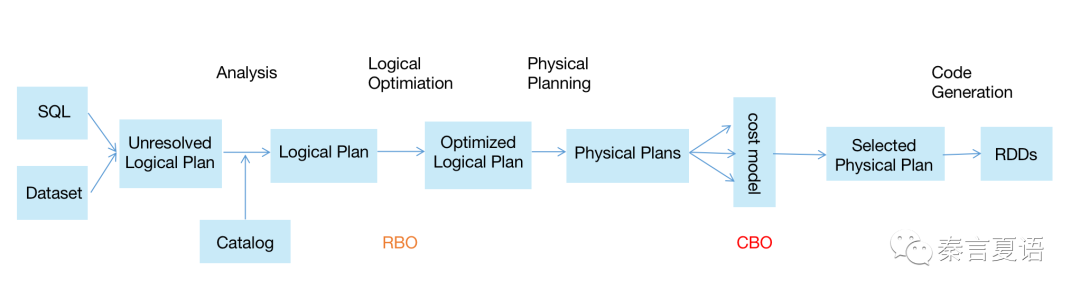Spark performs RBO first and then performs CBO.

It is also easy to understand:

• On the one hand, although RBO has shortcomings, it has many very definite and effective rules, such as Predicate Pushdown. It would be a pity if these rules are not used.
• On the other hand, CBO has premises and costs. If there is not enough statistical data to support it, you cannot make full use of CBO. At this time, it is necessary to have RBO to hold the bottom line of performance.

Therefore, RBO and CBO have become a coexistence relationship, each performing its duties to ensure SQL query performance.

## TL;DR

This article focuses on why there is a CBO and how it is implemented.

• It is difficult for RBO based on experience induction to cover all aspects. Thus, more direct performance optimization methods are required.
• New performance optimization methods should focus on CPU and IO consumption by abstracting resources.
• In addition to metrics, it is necessary to determine the calculation formula to easily compare consumption or cost. This optimization method is called CBO.
• The cost of an SQL statement can be accumulated layer by layer from AST, so the cost of each operation needs to be defined.
• Both Hive and Spark define their cost formulas, and the cost formulas evolve gradually and need to balance accuracy and cost. There is no perfect formula.
• CBO relies heavily on data statistical analysis and needs to run the analyze command to collect statistical data in advance.
• RBO and CBO are not substitutes but play their roles at different stages of query optimization.

Currently, mainstream databases, including traditional relational databases and distributed databases, basically use CBO as the main means to optimize SQL query performance. It improves the overall performance and reduces use costs.

However, CBO relies heavily on statistical data, and the collection and computing of these data are costly. It also faces the problem of not being updated in time, making it difficult for CBO to produce the desired effect.

The next article will discuss whether there is a more flexible method to optimize SQL query performance than CBO.

This is a carefully conceived series of 20-30 articles. I hope to give everyone a core grasp of the distributed system in a storytelling way. Stay tuned for the next one!

0 1 0
Share on

# Related Products

• ##Hybrid Cloud Distributed Storage

Provides scalable, distributed, and high-performance block storage and object storage services in a software-defined manner.

• ##Storage Capacity Unit

Plan and optimize your storage budget with flexible storage services

• ##Elastic Block Storage

Block-level data storage attached to ECS instances to achieve high performance, low latency, and high reliability

• ##Quick Starts

Deploy custom Alibaba Cloud solutions for business-critical scenarios with Quick Start templates.